## Wednesday, April 26, 2017

### Apothem

(Click on the comic if you can't see the full image.)(C)Copyright 2017, C. Burke.

I tried a rousing rendition of the Nation Apothem, but it didn't help.

Come back often for more funny math and geeky comics.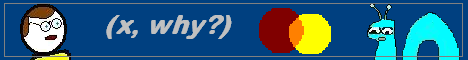## Saturday, April 22, 2017

### (x, why?) Mini: Scatter!

(Click on the comic if you can't see the full image.)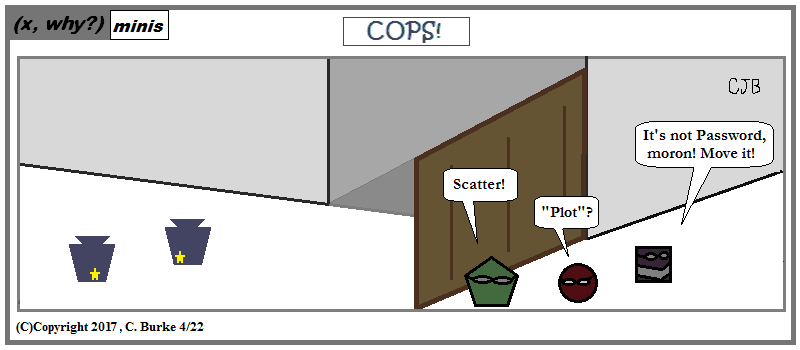(C)Copyright 2017, C. Burke.

Bad shapes! Bad shapes! What you gonna do...?

They're my keystones to comedy.

Come back often for more funny math and geeky comics.## Tuesday, April 18, 2017

### Geometry Problems of the Day

The following problems were taken from the GEOMETRY (COMMON CORE) Regents Exam given on Thursday, January 26, 2017.
Previous problems can be found here.

### Part 1

19. Parallelogram ABCD has coordinates A(0,7) and C(2,1). Which statement would prove that ABCD is a rhombus?

(3) The slope of BD is 1/3..

The diagonals of a rhombus are perpendicular. That means that the slopes of the diagonals are inverse reciprocals.
The slope of AC is (1 - 7) / (2 - 0) = -6 / 2 = -3.
Therefore, the slope of BD must be +1/3.

The midpoint of AC is (1, 4), which would be true regardless of the shape of the parallelogram. The length of diagonal BD is not restricted by the location of points A and C; the length of one diagonal does not affect the other. Finally, the slope of AC is NOT 1/3.

20. Point Q is on such that MQ:QN = 2:3. If M has coordinates (3,5) and N has coordinates (8,-5), the coordinates of Q are

(1) (5, 1)
2 + 3 = 5, so Q is 2/5 of the way from M to N.
(2/5)(8 - 3) = +2 and (2/5)(-5 -5) = -4.
The coordinates of Q are (3 + 2, 5 - 4), or Q(5, 1).

Continue to the next problems.

### Algebra 2 Problems of the Day

The following problems were taken from the ALGEBRA II (Common Core) Regents Exam given on Friday, January 27, 2017.
Previous problems can be found here

### Part 1

19. Which statement regarding the graphs of the functions below is untrue?

f(x) = 3 sin 2x, from -π < x < π
h(x) = log2x
g(x) = (x - 0.5)(x + 4)(x - 2)
j(x) = -|4x - 2| + 3 (1)

(2) f(x), h(x), and j(x) have one y-intercept.

All four are functions, so they have at most one y-intercept. However, the log function has a domain of positive numbers, x > 0, so it will not have a y-intercept.

20. When g(x) is divided by x + 4, the remainder is 0. Given g(x) = x4 + 3x3 - 6x2 - 6x + 8, which conclusion about g(x) is true? (1

(2) g(-4) = 0

The polynomial can be divided by x + 4 without a remainder, meaning (x + 4) is a factor. This makes -4 a zero of the function.

Continue to the next problems

## Monday, April 17, 2017

### Geometry Problems of the Day

The following problems were taken from the GEOMETRY (COMMON CORE) Regents Exam given on Thursday, January 26, 2017.
Previous problems can be found here.

### Part 1

17. Which rotation about its center will carry a regular decagon onto itself?

(4) 252°.

A full rotation is 360°. One-tenth of a rotation is 36°, and each one-tenth of a rotation will map a regular decagon onto itself.
Of the choices given, only 252° is a multiple of 36°.

18. The equation of a circle is x2 + y2 - 6y + 1 = 0. What are the coordinates of the center and the length of the radius of this circle?

(1) center (0,3) and radius 2(2)1/2
First, convert x2 + y2 - 6y + 1 = 0 to standard form by completing the square.
Half of -6 is -3, and (-3)2 = 9, so add 8 to both sides of the equation to increase 1 to 9.
So x2 + y2 - 6y + 9 = 8
Then x2 + (y - 3)2 = 8.
The coordinates of the vertex are (0, 3) and the radius is SQRT(8), which is 2(2)1/2

Continue to the next problems.

### Algebra 2 Problems of the Day

The following problems were taken from the ALGEBRA II (Common Core) Regents Exam given on Friday, January 27, 2017.
Previous problems can be found here

### Part 1

17. What is the solution, if any, of the equation

2 / (x + 3) - 3 / (4 - x) = (2x - 2) / (x2 - x - 12)

(1) -1

Follow along with the image below.
Because x2 - x - 12 is the product of (x + 3)(x - 4), we can multiply the entire equation by (x + 3)(x - 4).
To get (x - 4) in the denominator of the middle fraction, multiply the top and bottom of the fraction by -1.
Multiplying removes all the denominators and sets up an equation that solved in a couple of steps.
The solution is x = -1.

Because it was multiple choice, you could also use the graphing calculator to solve the problem:
As shown below, put the left side of the equation into y1 and the right side into y2 and graph.
Check the values for -1 and -5. You can see that -1 has the same values but -5 does not. Likewise, all real numbers and no real solutions are obviously incorrect.

18. In 2013, approximately 1.6 million students took the Critical Reading portion of the SAT exam. The mean score, the modal score, and the standard deviation were calculated to be 496, 430, and 115, respectively.
Which interval reflects 95% of the Critical Reading scores?

(4) 496 + 230

The standard deviation uses the mean, which is 496 in this example. About 95% of the data falls within TWO standard deviations from the mean. Double 115 and you get 230.

Continue to the next problems

## Sunday, April 16, 2017

### Happy Easter 2017!

(Click on the comic if you can't see the full image.)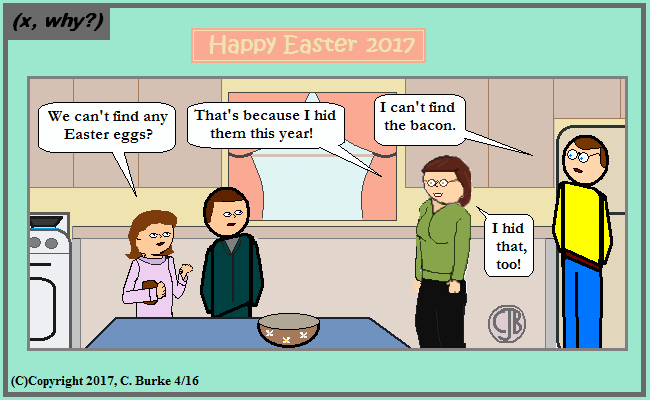(C)Copyright 2017, C. Burke.

Hope your hunts are fun! (But hiding bacon is just mean, whatever the doctors say.)

Come back often for more funny math and geeky comics.## Saturday, April 15, 2017

### Algebra 2 Problems of the Day

The following problems were taken from the ALGEBRA II (Common Core) Regents Exam given on Friday, January 27, 2017.
Previous problems can be found here

### Part 1

15. The loudness of sound is measured in units called decibels (dB).
These units are measured by first assigning an intensity I0 to a very softsound that is called the threshold sound. The sound to be measured is assigned an intensity, I, and the decibel rating, d, of this sound is found using d = 10 log (I / I0).
The threshold sound audible to the average person is 1.0 X 10-12 W/m2 (watts per square meter).
Consider the following sound level classifications:

How would a sound with intensity 6.3 X 10-3 W/m2 be classified?
 Moderate 45-69 dB Loud 70-89 dB Very Loud 90-109 dB Defeaning > 110 dB

(3) very loud

Don't let all the logs and subscripts bother you. At the heart of this question is simple substitution and evaluation. Plug in the values and let the calculator do the rest.
The formula you are given is: d = 10 log (I / I0).
I0 = 1.0 X 10-12, and I = 6.3 X 10-3
So calculate (using enough parentheses!) the following: 10 log ( (6.3 X 10-3) / (1.0 X 10-12) )
You should get 97.99341..., which is between 90 and 109, or "Very Loud".

16. Pedro and Bobby each own an ant farm. Pedro starts with 100 ants and says his farm is growing exponentially at a rate of 15% per month. Bobby starts with 350 ants and says his farm is steadily decreasing by 5 ants per month.
Assuming both boys are accurate in describing the population of their ant farms, after how many months will they both have approximately the same number of ants?

(2) 8

The equation for Pedro's farm is y = 100(1.15)x. The equation for Bobby's farm is y = 350 - 5x. (Note that Bobby is losing 5 ants, not 5%.)
Graph these on your calculator.
The two lines will intersect at a fraction past 8 months, where both will have a little less than 310 ants.
Use the CALC function (2nd and TRACE) and choose Intersection from the menu. Hit ENTER three times to get the answer.

Continue to the next problems

### Geometry Problems of the Day

The following problems were taken from the GEOMETRY (COMMON CORE) Regents Exam given on Thursday, January 26, 2017.
Previous problems can be found here.

### Part 1

15. In circle O, secants ADB and AEC are drawn from external point A such that points D, B, E, and C are on circle O. If AD = 8, AE = 6, and EC is 12 more than BD, the length of BD is

(2) 22.

I sketched the image before solving so it isn't drawn to scale. This is a problem that you might want to work backward from the answers, plugging numbers into the equations.

The rule to remember is that (AD)(AB) = (AE)(AC)
or (8)(x + 8) = (6)(x + 12 + 6)
Simplify: 8x + 64 = 6x + 108
2x = 44
x = 22

Check: (8)(22 + 8) = (6)(22 + 12 + 6)
(8)(30) = (6)(40)
240 = 240

16. A parallelogram is always a rectangle if

(1) the diagonals are congruent
Congruent diagonals is true of rectangles and isosceles trapezoids (which aren't parallelograms, so they don't count here).
Opposite angles congruent and diagonals bisecting each other are true of all parallelograms, not just rectangles.
Diagonals intersecting at right angles proves that the quadrilateral is a rhombus, not a rectangle.

Continue to the next problems.

## Friday, April 14, 2017

### Algebra 2 Problems of the Day

The following problems were taken from the ALGEBRA II (Common Core) Regents Exam given on Friday, January 27, 2017.
Previous problems can be found here

### Part 1

13. The price of a postage stamp in the years since the end of World War I is shown in the scatterplot below.

The equation that best models the price, in cents, of a postage stamp based on these data is

(3) y = 1.43(1.04)x

Looking at the graph, you can see that it has an exponential shape, starting somewhat flat and then increasing more quickly. This eliminates choices (1) and (4). (And, really, a sine graph would be a silly choice here.) If you put the other two into your graphing calculator and expand the size of the window, you will see that choice (2) increases much more quickly than the graph in the question. Choice (3) is a better fit.
If you have a problem expanding the window size, you can also check the Tables of Values to see that (2) shoots up to quickly.

14. The eighth and tenth terms of a sequence are 64 and 100. If the computations. sequence is either arithmetic or geometric, the ninth term can not be

(1) -82

In an arithmetic sequence, the common difference can be found be subtracting the 8th term from the 10th term and dividing by 2 (which is 10 - 8). If we do that, we get (100-64)/2, which is 18.
64 + 18 = 82, which eliminates choice (4). (At this point, you might suspect that -82 is likely the answer.)
In a geometric sequence, the common ratio can be found by dividing the 10th term by the 8th term and then taking the square root (because the ratio is applied twice to get from the 8th term to the 10th term).
So (100/64)^.5 = +1.25, which are the common ratios. It is important to remember that the square root may positive or negative.
Then 64 * 1.25 = 80, and 80 * 1.25 = 100
And 64 * (-1.25) = -80 and -80 * 1.25 = 100.
This eliminates choices (2) and (3).

Continue to the next problems

### Geometry Problems of the Day

The following problems were taken from the GEOMETRY (COMMON CORE) Regents Exam given on Thursday, January 26, 2017.
Previous problems can be found here.

### Part 1

13. On the graph below, point A(3,4) and with coordinates B(4,3) and C(2,1) are graphed. What are the coordinates of B' and C' after undergoes a dilation centered at point A with a scale factor of 2?

(1) B'(5,2) and C'(1,-2) .
A dilation with scale factor 2 centered at A means that everything will be twice as far from A as it currently is.
Point B is 1 unit right and 1 unit down from A, so B' will be 2 units right and 2 units down from A, or (5, 2).
Point C is 1 unit left and 3 units down from A, so C' will be 2 units left and 6 units down from A, or (1, -2).

14. In the diagram of right triangle ADE below, BC || DE.

Which ratio is always equivalent to the sine of ∠A?

(3) BC / AB
Sine = Opposite / Hypotenuse. There are two right triangles containing Angle A. The sides opposite angle A are BC in the smaller triangle and DE in the bigger triangle. Note that because BC || DE, Angle ACB must be a right angle because it corresponds to Angle E. The hypotenuse of the smaller triangle is AB, and the hypotenuse of the bigger triangle is AD.
The two possible answers to this question, therefore, are BC / AB, and DE / AD. The answer is choice (3).

Continue to the next problems.

## Thursday, April 13, 2017

### Algebra 2 Problems of the Day

The following problems were taken from the ALGEBRA II (Common Core) Regents Exam given on Friday, January 27, 2017.
Previous problems can be found here

### Part 1

11. The solution to the equation 18x2 - 24x + 87 = 0 is

(4) (see image)

Follow along with the image. First, note that all the numbers are multiples of 3, so we can factor the equation by 3, allowing us to deal with some smaller numbers.
This makes a = 6, b = -8 and c = 29. You can do this with the larger numbers, too, and you should get the same answer.
Substitute the numbers into the Quadratic Formula and simplify, using the Order of Operations.
Once you get the discriminant = -632, you know that you have an imaginary answer. From the choices, you know that you will have 158 in the answer, and 632 / 158 = 4, which is a perfect square.
Note: At this point, you don't actually have to solve this. As you continue, you will likely be able to eliminate the three wrong choices. You can see that (8 + 2i) / 12 can only reduce to choice (4).

12. When g(x) = 2 / (x + 2) and h(x) = log(x + 1) + 3 are graphed on the same set of axes, which coordinates best approximate their point of intersection?

(2) (-0.9,1.9)
Put the equations into the graphing calculator and hit Graph. Next use "2nd" and "Trace" to get the Calc menu.
Select option 5, Intersection. Hit "ENTER" three times.
The x-coordinate is approximately -0.9269206, which rounds to -0.9.
The y-coordinate is approximately 1.863795, which rounds up to 1.9.

Continue to the next problems

### Geometry Problems of the Day

The following problems were taken from the GEOMETRY (COMMON CORE) Regents Exam given on Thursday, January 26, 2017.
Previous problems can be found here.

### Part 1

11. A solid metal prism has a rectangular base with sides of 4 inches and 6 inches, and a height of 4 inches. A hole in the shape of a cylinder, with a radius of 1 inch, is drilled through the entire length of the rectangular prism.

What is the approximate volume of the remaining solid, in cubic inches?

(2) 77.
The Volume of the prism is 4 x 4 x 6 or 96 cubic inches. The Volume lost by drilling the cylinder is (1)2(pi)(6), which is approximately 18.85. Subtract 96 - 19 = 77.

12. Given the right triangle in the diagram below, what is the value of x, to the nearest foot?

(3) 18
Cos (40) = 14 / x.
so x = 14 / cos (40) = 18.3
Make sure your calculator is in Degree mode.

Continue to the next problems.

### Hole Numbers

(Click on the comic if you can't see the full image.)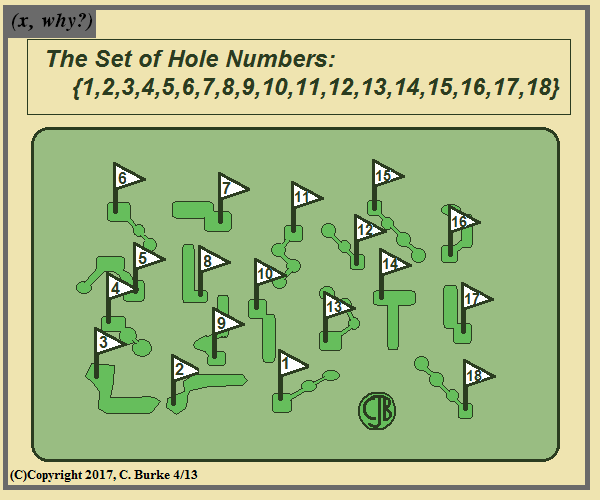(C)Copyright 2017, C. Burke.

I guess the driving range would be 0, and 19 is, of course, the bar.

Come back often for more funny math and geeky comics.## Monday, April 10, 2017

### (x, why?) Mini: Ain't That Right?

(Click on the comic if you can't see the full image.)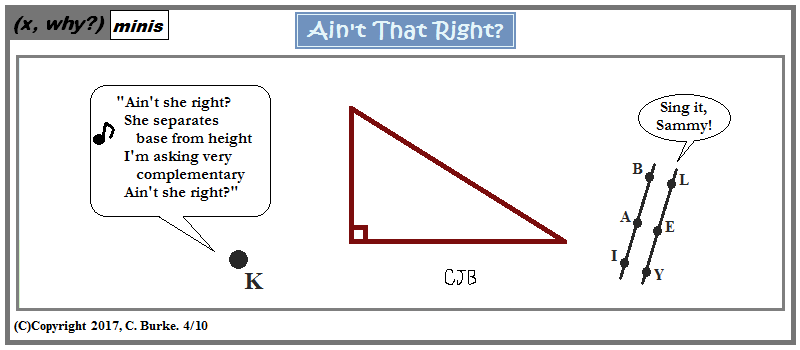(C)Copyright 2017, C. Burke.

This is one of those times a ''mini'' nearly blew up because of the choices for verses that started to occur to me.

Come back often for more funny math and geeky comics.## Thursday, April 06, 2017

### (x, why?) Mini: What's True?

(Click on the comic if you can't see the full image.)(C)Copyright 2017, C. Burke.

Math. Math is to say what's true. It's called Logic.

Come back often for more funny math and geeky comics.## Wednesday, April 05, 2017

### Findings

(Click on the comic if you can't see the full image.)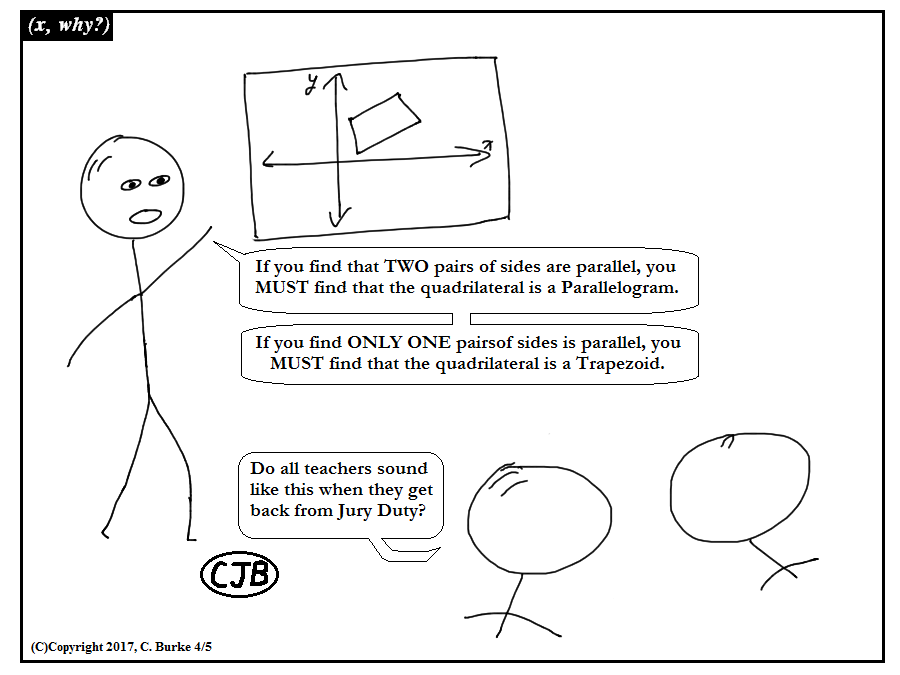(C)Copyright 2017, C. Burke.

If your quadrilateral takes flight, it may be a kite.

Come back often for more funny math and geeky comics.